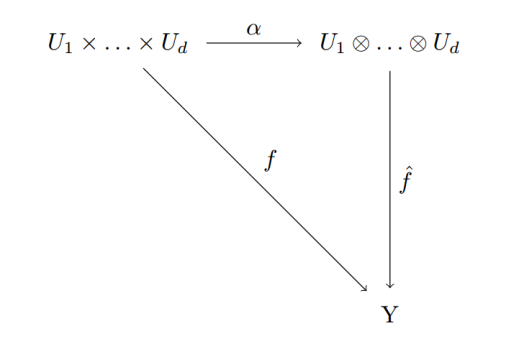Category Theory

# Products in a Category – Exercise 1Demonstrate that A × B ≅ B × A.

Solution:
The product A × B satisfies the universal property, for any object X and arrows r: X→A and s: X→B :

there existis only one arrow f: X → A × B such that $$\pi_{A}\circ f = r$$ and $$\pi_{B}\circ f = s$$, where πA : A × B → A and πB :A × B → B.

Let $$X=B\times A$$, $$r = \tilde{\pi}_{A}: B\times A \longrightarrow A$$ and $$s = \tilde{\pi}_{A}: B\times A \longrightarrow B$$, we obtain unique arrows f: B × A → A × B and g: A × B → B × A with the following properties:

• $$\pi_{A}\circ f = \tilde{\pi}_{A}$$;
• $$\tilde{\pi}_{A}\circ g = \pi_{A}$$.

Thus, we have $$\pi_{A}=\pi_{A}\circ (f\circ g)$$. (We have the same for  πB). Clearly, $$I_{A\times B} = f\circ g$$, but, since there exist only the arrows f and g satisfying the property, we have no choice but $$I_{A\times B} = f\circ g$$.

Applying the same property for $$\tilde{\pi}_{A}=\tilde{\pi}_{A}\circ (g\circ f)$$, we have only one choice: $$g\circ f = I_{B\times A}$$.

Tags: